In mathematics, we sometimes calculate the length of the arc and the area of a sector. How do we calculate an arc length and a sector area?

When calculating the arc length and the sector area, we must understand the properties of a circle. If we can calculate the length of the circumference and the area of the circle, we can get the arc length and the sector area. Since the calculation of the circle is essential, we must use the pi.

The problem of finding the arc length and the sector area is an advanced problem of circumference and area of a circle.

If you have memorized the formulas for finding the circumference and area of a circle, it is not difficult to find the arc length and the sector area. Also, you don’t need to learn any new formulas. We will explain how to find the arc length and the sector area.

## In the Formula for Diameter and Area of a Circle, Let Pi to $π$

In order to get the arc length and the sector area, we must get the circumference and the area of the circle before we calculate. So, let’s review the calculation method.

For the circumference and area, we can calculate the following formulas.

• Circumference = Diameter × 3.14 (pi)
• Area of a circle = Radius × Radius × 3.14 (pi)

In advanced mathematics, we don’t use 3.14 as pi. This is because 3.14 is not an exact number but only an approximation. Instead, we use the symbol $π$. $π$ means pi. This is easier to calculate because we can omit the multiplication by 3.14.

In math, we do calculations that use letters in algebraic expressions. So instead of multiplying by 3.14, we replace pi with the letter $π$. Thus, we have the following formula.

• Circumference = Diameter × $π$
• Area of a circle = Radius × Radius × $π$Since $π$ is the pi, you can calculate it by thinking of $π=3.14$ in math. You can use $π$ or you can multiply by 3.14. Both are correct, but if you’ve learned algebraic expressions in math, you should use $π$ for pi.

### Pi is One of the Definitions

Why do we need to multiply the diameter by pi to get the circumference of a circle? It is because it is defined as such.

After measuring the length of the circumference, we measured the radius of the circle, and it happened to be about 3.14. So we defined this number as pi. Pi is a definition. It is defined that multiplying the diameter by pi to get the length of the circumference.

Therefore, it doesn’t make sense to ask, “Why is the diameter multiplied by pi to get the circumference length?” Pi is a definition, and it just happens to be the number about 3.14.

The same can be said for the area of a circle. Pi is a definition, so try to remember the formula when figuring out the circumference and area of a circle.

## The Arc Length and the Sector Area Has a Formula Using the Central Angle

We’ve reviewed the area of a circle. Why is it important to review the circumference and area of a circle before learning about the arc length and the sector area? It’s because if we don’t understand the circumference and area of a circle, it’s impossible to find the arc length and the sector area.

The circumference part of a sector is called an arc. Also, the angle enclosed by the arc and the two radiuses is called the central angle.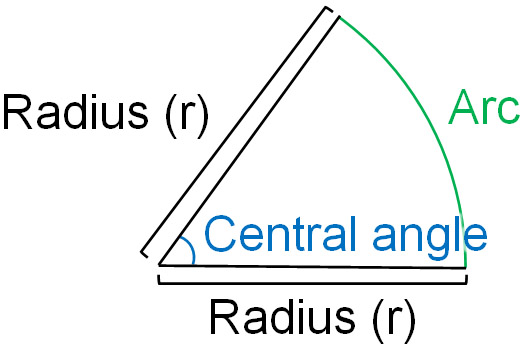Part of the circle is a sector. We need to be able to answer which part of the sector is the arc and the central angle.

If we understand these words and have learned the formulas for circumference length and area of a circle, the way to find the arc length and the sector area is simple. There is no need to learn a new formula for arc length and sector area.

### You Can Calculate the Arc Length from the Center Angle

How do we calculate the arc length of a sector? There is a formula for the arc length as follows.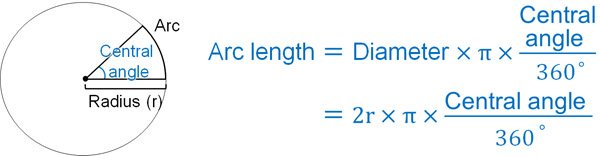Compared to the formula for circumference, $\displaystyle\frac{central~angle}{360°}$ is added. This is the formula for finding the circumference length.

However, as mentioned above, we don’t need to remember it. Even if we don’t remember this formula, it’s obvious. The total angle of a circle is 360 degrees. Therefore, the length of the arc varies depending on the size of the central angle.

For example, suppose we have a circular cake. If this cake is divided into halves, the central angle is 180°. If the cake is divided into thirds, the central angle is 120°.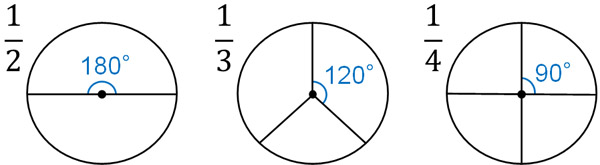What is the length of the arc? If you divide it into two, the central angle is halved, and at the same time, the length of the arc is reduced by half. If it is divided into three pieces, the central angle will be $\displaystyle\frac{1}{3}$, and the arc length will be $\displaystyle\frac{1}{3}$.

In the sector shape, as the central angle decreases, the length of the arc decreases in the same proportion. So we first determine the circumference length using the formula and then multiply it by $\displaystyle\frac{central~angle}{360°}$. As a result, we can find the arc length of a sector.

### Find the Area of a Sector from an Angle

In the same way, we can find the area of a sector. In other words, we first calculate the area of the circle. Then, calculate the area of the sector according to the size of the central angle. There is a formula for finding the area of a sector, which is as follows.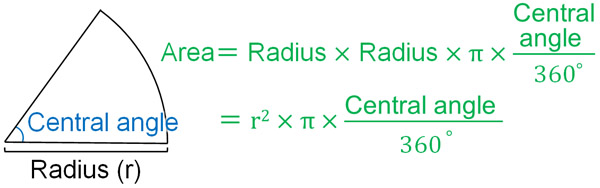The angle of a circle is 360°. Therefore, if the central angle becomes one-half, and at 180°, the area of the circle will be one-half. The idea is the same as the arc length of a sector.

Likewise, if the central angle of the circle becomes one-third (120°), the area of the circle becomes one-third. If the center angle becomes one-fourth (90°), the area is reduced to one-fourth. If the central angle becomes smaller, the sector area will be correspondingly smaller.

Thus, multiplying the area of a circle by $\displaystyle\frac{central~angle}{360°}$ yields the area of a sector.

This is why we don’t need to remember the formula for finding the arc length and the sector area. If we remember the formulas for finding the length of the circumference and the area of the circle, we can find the arc length and the sector area. After calculating the circumference and area of the circle, multiply it by the proportion of the central angle to get the arc length and the sector area.

### From the Arc Length and the Sector Area, Calculate the Central Angle

Once we understand these details, we can also calculate the central angle of a sector. Even if we don’t know the central angle, if we know the arc length or the sector area, we can find the central angle. For example, how is the following problem?

• Find the central angle of a sector with an arc length of $4π$ cm and a radius of 3 cm.

We already know the arc length. In this case, if the central angle is $x$, we can create the following equation.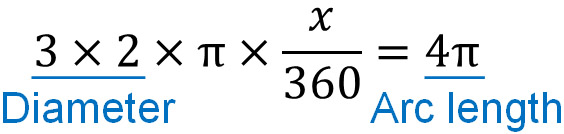Then solve this equation.

$3×2×π×\displaystyle\frac{x}{360}=4π$

$6π×\displaystyle\frac{x}{360}=4π$

$x=4π×360×\displaystyle\frac{1}{6π}$

$x=240$

Thus, we can calculate that the central angle is 240°.

## Exercises: Calculate the Arc Length and the Sector Area

Q1: Solve the following problem.

For a sector with a radius of 6 cm and an arc length of $2π$, find the central angle and area.

First, calculate the central angle. If the central angle is $x$, we have the following equation.

• $6×2×π×\displaystyle\frac{x}{360}=2π$

Doing this calculation, we get the following.

$6×2×π×\displaystyle\frac{x}{360}=2π$

$12π×\displaystyle\frac{x}{360}=2π$

$x=2π×360×\displaystyle\frac{1}{12π}$

$x=60$

The central angle is 60°. Once we know the central angle, we can find the area of the circle. Substituting into the formula for the area of a sector, we get the following.

• $6×6×π×\displaystyle\frac{60}{360}=6π$

Therefore, the area of a sector is $6π$.

Q2: Solve the following problem.

There are two sectors in the square, as shown below. Let’s calculate the area of the shadow.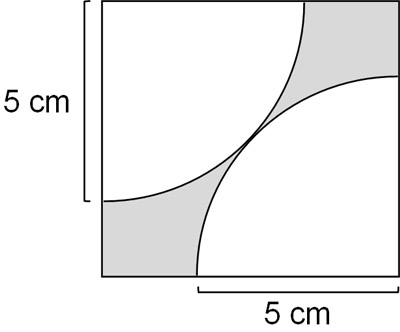In advanced sector problems, they are often presented in combination with other shapes. In this problem, it is a combination of a square and sectors. To get the shadow area, we need to use two shapes. Specifically, we can see that by subtracting two sectors from the square, we can get the shadow area.

• Shadow area = Square area – Sector area

First, let’s get the area of a sector. There are sectors in a square, and a sector has a central angle of 90°. Therefore, one sector area can be calculated as follows.

• $5×5×π×\displaystyle\frac{90}{360}=\displaystyle\frac{25}{4}π$

Since it contains two sectors, the total area is as follows.

• $\displaystyle\frac{25}{4}π×2=\displaystyle\frac{25}{2}π$

On the other hand, how do we get the area of the square? If we consider the following, we can see that the diagonal is 10 cm.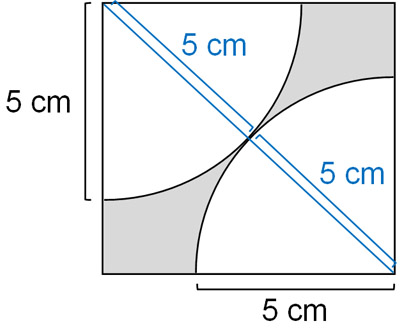If we know the diagonals, we can get the area of a square. A square is the same as a rhombus. The area of a rhombus can be found by the following formula.

• Diagonal length × Diagonal length ÷ 2

In the case of a square (rhombus), we can consider the following figure.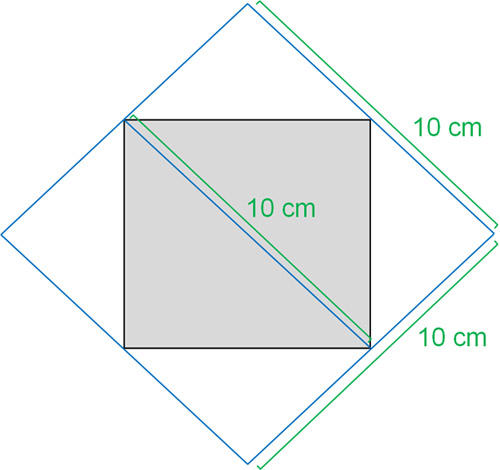If the diagonal of a square is 10 cm, then the length of the vertical and horizontal sides of the square surrounding it will be 10 cm each. Therefore, we can get the area of the larger square by multiplying the length of the diagonals. However, since we want to calculate the rhombus (the smaller square), the area is half.

So, we have the following calculation.

• $10×10×\displaystyle\frac{1}{2}=50$

The area of the square is 50 cm2, and the total area of sectors is $\displaystyle\frac{25}{2}π$ cm2. Therefore, the area of the shadow is $50-\displaystyle\frac{25}{2}π$ cm2.

## Learn How to Find the Arc Length and the Sector Area

In mathematics, we will learn about sectors. We need to be able to calculate the arc length and the sector area.

If we remember the formulas for the circumference and area of a circle, it is easy to find the arc length and the sector area. After calculating the circumference and area of the circle, we can reduce the number according to the rate of the central angle. The angle of the circle is 360°, and the smaller the central angle, the smaller the arc length and the sector area at the same ratio.

Pi is calculated to be 3.14. On the other hand, in mathematics, after learning the algebraic equation, pi is set to $π$. The concept is the same, so it doesn’t matter which way you calculate it. Of course, the calculation using $π$ is more likely to result in fewer miscalculations because you can omit the multiplication by 3.14.

In this way, we can find the arc length and the sector area. Since the advanced problems are combined with other shapes, understanding the features of the other shapes will help you solve the problems.